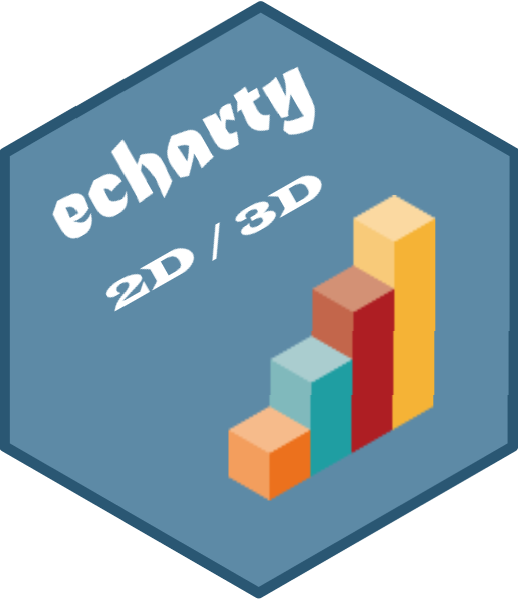# echarty

Minimal R/Shiny Interface to ECharts.js

# Use Case 4 - Sankey with timeline

Will sankey chart work with timeline ? An interesting question by rdatasculptor.
To speed things up, we go to echarty’s examples. In the Console type ?ec.examples, then in Help panel, hit Ctrl/F and seach for ‘sankey’. We find the following code

``````#------ Sankey and graph plots
sankey <- data.frame(
node   = c("a","b", "c", "d", "e"),
source = c("a", "b", "c", "d", "c"),
target = c("b", "c", "d", "e", "e"),
value  = c(5, 6, 2, 8, 13)
)
data <- ec.data(sankey, 'names')

ec.init(preset= FALSE,
series= list(list(
type= 'sankey',
data= lapply(data, function(x) list(name= x\$node)),
edges= data ))
)
``````

Sankey chart data consists of nodes connected by edges having a value. If the data is defined as data.frame, we need to transform it into a list with command ec.data(). Once in that format, we can access the columns by index - node is value, …, value is value. The edges definition requires three parameters - source, target and value.

Now let’s add timeline. Timeline is just a collection of data states targeted to some chart. An animated display shows the chart transition from one state to the next.
We will not try to change nodes or edges on each step, just edge values. Let’s have three states(steps) and build three edge lists with slightly different values - edges1, edges2, edges3. That wraps up the data preparation.

The GUI part starts with chart initialization with ec.init(), then setting chart parameters which here are series, timeline and options.
Parameter timeline defines labels for the timeline legend.
Parameter options (ill-named) defines the timeline series.
Note: command ec.init can set timeline options through parameter tl.series, but that requires grouped dataframe data, which is not the case in this example.
The complete code is below. We’ve added also series levels to fine-tune visuals on each step, especially colors. Without levels node colors would change on each step, which is confusing.

``````sankey <- data.frame(
node = c("a","b", "c", "d", "e"),
source = c("a", "b", "c", "d", "c"),
target = c("b", "c", "d", "e", "e"),
value = c(5, 3, 2, 8, 13)
)
# prepare timeline state data
st <- function() ec.data(sankey, 'values')  # data.frame to list
nodes <- lapply(st(), function(x) list(name = x\$value))
edo <- function(x) list(source=as.character(x\$value),
target=as.character(x\$value), value=x\$value)
edges <- list()
edges[] <- lapply(st(), edo)
sankey\$value <- c(4, 5, 4, 7, 8)
edges[] <- lapply(st(), edo)
sankey\$value <- c(2, 7, 6, 5, 4)
edges[] <- lapply(st(), edo)

options <- list()
for(i in 1:3) {
options <- append(options,
list(list(title = list(text=paste0('step',i)),
series= list(list(type='sankey', data=nodes, edges=edges[[i]]))))
)
}
# optional serie levels to keep colors persistent
i <- -1
levcol = lapply(list('blue','red','green','brown','yellow'),
function(clr) { i<<-i+1; list(depth=i, itemStyle=list(color=clr)) })

ec.init(preset= FALSE,
series = list(list(type='sankey', data = nodes, edges = edges[], levels = levcol	)),
timeline = list(axisType='category', data=list('s1','s2','s3')),
options = options
)
``````So the final answer is yes - sankey and timeline work together just fine.
For more sankey customizations check this ECharts example.